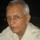Coffee Room
Discuss anything here - everything that you wish to discuss with fellow engineers.
12829 Members
Join this group to post and comment.bobgalle • Oct 13, 2015

# moving average calculation

how to do moving average calculation, for ex: i am getting continuous values from a (analog/ real/eg. 12.125, 3.75 ton/hour)flow transmitter. i need to know the average of the values that i am getting from the transmitter every sec.. i need a moving avg of say 100sec... which means at any time if i take 100sec i need the avg.What is said next is a trivial solution. No idea how it can be implemented.
Consider two registers.
The first one stores the flow rate at the end of each second, replacing the value of the previous second.
The second accumulates the values for 100 seconds. At the end of 100 seconds the accumulated value is 100 times the average. Just shift the out put by two decimal places to the left.
After the 100th second add the value of the next second and subtract the value in the first register. You will now have the average flow between the first and 101st second and so on.bobgalle • Oct 14, 2015
hmmm i agree... but totally i will end up doing 100 registers (second register capturing the previous sec value means at the 3 sec itself i will loose first one)... the amount of registers i use gets creepier in the code.

is there any solution using arrays??